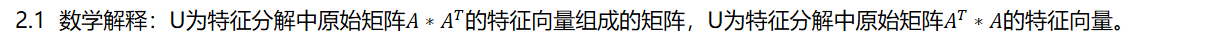# 【ML-14-2】奇异分解(SVD) 案例--用户与商家稀疏矩阵## 一、稀疏矩阵(sparse matrix):

### 1.2 数据构建：

500样本数由来：使用随机函数确定50*200的随机坐标。

spr_A=sparse.coo_matrix((data, (row, col)), shape=(50, 200)).toarray()

## 二、U和V的矩阵的含义：

### 假设原始矩阵为：A,分解后的矩阵为A=U*S*V2.2 题目解释：U表示user-to-concept的相似矩阵，每个列向量表示用于的评分高低的特征。V表示shop-to-concept的相似矩阵，转置后每个行向量表示商家本身服务质量。

## 三、代码

```# Author:yifan
from scipy import sparse
import numpy as np
import  xlwt
from numpy import linalg as la

col=*500
row=*500
data=*500
for i in range(500):
row[i]=np.random.randint(0,50)
col[i]=np.random.randint(0,200)    #返回值的范围为【0，200)的整数,200不可取，因为是矩阵的index最大为199
data[i]=np.random.randint(1,6)     #返回值的范围为【1，5】的整数，作为评分
# print(max(col))
spr_A=sparse.coo_matrix((data, (row, col)), shape=(50, 200)).toarray()   #构造50*200的矩阵
# print(spr_A)
# 创建工作薄，并保存上述矩阵
f = xlwt.Workbook()
sheet1 = f.add_sheet(u'sheet1', cell_overwrite_ok=True)  # 创建sheet
for c in range(200):
for r in range(50):
sheet1.write(r,c,int(spr_A[r,c]))   #注意要使用int(spr_A[r,c])，直接使用spr_A[r,c]会报错
f.save('D:/excelText.xls')

print(spr_A.shape)
#SVD变换：
u,s,v = la.svd(spr_A,full_matrices=0,compute_uv=1)
#还原原始的矩阵，发现四OK的
# A=np.dot(u,np.diag(s))
# print(np.dot(A,v))
print("左奇异值：")
print(u,u.shape)
print("奇异值：")
print(s,s.shape)
print("右奇异值：")
print(v,v.shape)
```

## 语法注解：

a是一个形如(M,N)矩阵

full_matrices的取值是为0或者1，默认值为1，这时u的大小为(M,M)，v的大小为(N,N) 。否则u的大小为(M,K)，v的大小为(K,N) ，K=min(M,N)。

compute_uv的取值是为0或者1，默认值为1，表示计算u,s,v。为0的时候只计算s。

u大小为(M,M)，s大小为(M,N)，v大小为(N,N)。

A = u*s*v

## 机器学习之正则化（Regularization）

### 微信扫一扫Close